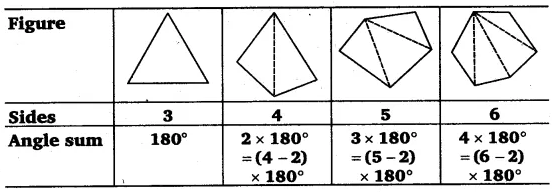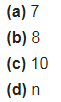# Examine the table. (Each figure is divided into triangles and the sum of the angles deduced from that.)

Examine the table. (Each figure is divided into triangles and the sum of the angles deduced from that.)What can you say about the angle sum of a convex polygon with number of sides?